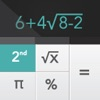Star Rating 70.0%

Votes

2

Version 2.5.7

Content Rating 4+

# Scientific Calculator +

## Sarah Ragab

### Utilities, Productivity

IOS
AUD 14.99

Scientific Calculator+ is an iPhone/iPad calculator designed with simplicity, usability, and beauty in mind...

Once you try Scientific Calculator+ you will think why this wasn't done from so long.

After installing Scientific Calculator+ you get access to 4 different calculators:
1- Handwriting calculator
2- Scientific calculator
3- Quadratic & Cubic equation solver
4- System of linear equations solver
5- Hex, Oct, Bin converter
6- Graphing calculator (you will need to upgrade to use this calculator)

FEATURES:
- Elegant & intuitive interface
- Supports handwriting
- Displays both the equation & the result at the same time
- Supports many scientific functions
- Solving system of linear equations
2x – y = 9
3x + 4y = –14
- Solving Quadratic equation
x² + 5x + 6 = 0
- Solving Cubic equation
2x³ - 4x² - 22x + 24 = 0
- Graphing equations

Note: for graphing equations you will need to upgrade to Graphing Calculator+, this will cost you extra \$0.99

Release Notes

Bug fixes and performance improvements© 2016 - 2020 apps-trader.com · All Rights Reserved.
Authorised iTunes affilate, all apps are downloaded from iTunes, all copyrights and trademarks remain the property of the original owners, E & O Accepted